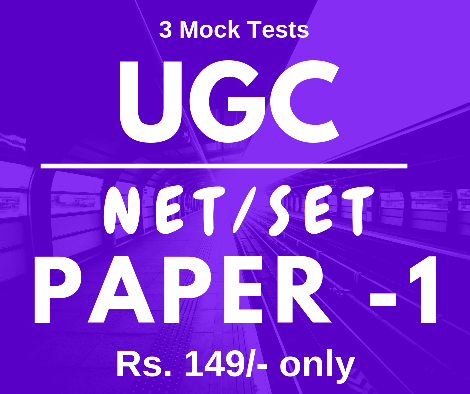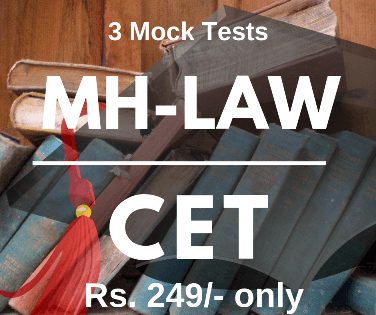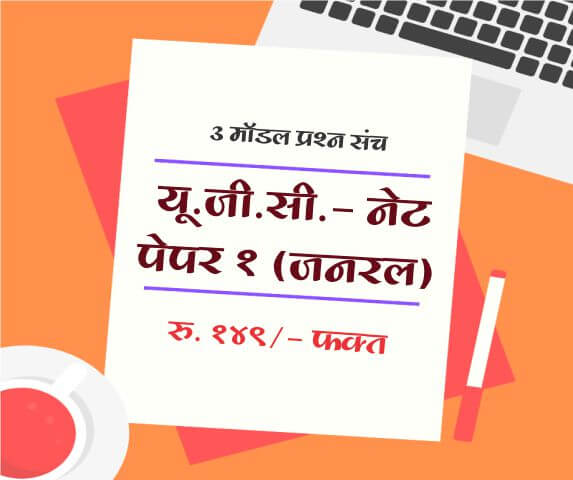# Trigonometric functions

The secondary trigonometric functions are the sine and cosine of an angle. These are sometimes abbreviated sin(θ) and cos(θ), respectively, where θ is the angle, but the parentheses around the angle are often omitted, e.g., sin θ and cos θ.

When the trigonometric functions are reflected from certain angles, the result is often one of the other trigonometric functions.

Trigonometric functions are as given below

1. $$sin (A + B) = sin A cos B + cos A sin B$$
2. $$sin (A - B) = sinA cosB - cos A sin B$$
3. $$cos (A + B) = cos A cos B - sin A sin B$$
4. $$cos (A - B) = cos A cos B + sin A sin B$$
5. $$tan (A + B) = {tan A + tan B \over 1 - tan A tanh B}.$$
6. $$tan (A - B) = {tan A - tan B \over 1 + tan A tanh B}.$$
7. $$sin (A + B) sin (A - B) = sin^2A - sin^2B$$
8. $$cos (A + B) cos (A - B) = cos^2 A - sin^2 B$$
9. $$2sin A cos B = sin (A + B) + sin (A - B)$$
10. $$2cos A sin B = sin (A + B) - sin (A - B)$$
11. $$2cos A cos B = cos (A + B) + cos (A - B)$$
12. $$2sin A sin B = cos (A - B) - cos (A + B)$$
13. $$sin C + sin D = 2sin ({C + D \over 2}) cos ({C - D \over 2})$$
14. $$sin C - sin D = 2cos ({C + D \over 2}) sin ({C - D \over 2})$$
15. $$cos C - cos D = 2cos ({C + D \over 2}) cos ({C - D \over 2})$$
16. $$cos C - cos D = - 2sin ({C + D \over 2}) sin ({C - D \over 2})...... (C > D)$$
OR
$$cos C - cos D = - 2sin ({C + D \over 2}) sin ({D - C \over 2})...... (C < D)$$
17. $$sin ({π\over 2} - θ) = cos θ$$
18. $$tan ({π\over 2} + θ) = - cot θ$$
19. $$cos ({π\over 2} - θ) = sin θ$$
20. $$cot ({π\over 2} - θ) = - tan θ$$
21. $$cosec ({π\over 2} - θ) = sec θ$$
22. $$sec ({π\over 2} - θ) = cosec θ$$
23. $$tan ({π\over 2} - θ) = cot θ$$
24. $$cot ({π\over 2} - θ) = tan θ$$
25. $$sin ({π\over 2} + θ) = cos θ$$
26. $$cos ({π\over 2} + θ) = - sin θ$$
27. $$cosec ({π\over 2} + θ) = sec θ$$
28. $$sec ({π\over 2} + θ) = - cosec θ$$

Labels: MPSC, TET, NET-SET, Maths, Mathamatics, Trignometric Functions

#### Articles

 Name : Comment :
Upcoming Exam Forms

Full Length Mock Tests
Timer Based Exams
Instant Result and assesment
Detailed analasys of Result##### UGC NET SET Paper 1 Mock Tests

UGC-NET / SET Paper 1 mock test

3 Mock Tests, 150 Questions with Explanations

Rs: 149 Only/-##### LAW MH-CET 2019 (3 YEAR COURSE)

Mock test for MH CET Law 2019 for three year law programme

3 Mock Tests, 450 Questions with Explanations

Rs: 249 Only/-##### यू. जी. सी. - नेट पेपर १ (जनरल)

यू. जी. सी. - नेट पेपर १ (अनिवार्य) सामान्य प्रश्न हिंदीमें

3 Mock Tests, 150 Questions with Explanations

Rs: 149 Only/-